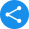# INMO 2020 Syllabus

## What is INMO?

The Indian National Mathematical Olympiad abbreviated as INMO is a high school mathematics competition. It is conducted annually in India. It is the second step for the International Mathematical Olympiad. INMO is conducted by the Homi Bhabha Centre for Science Education (HBCSE) on the behalf of the National Board of Higher Mathematics (NBHM). The INMO Syllabus is pre-degree college mathematics. The INMO exam paper comprises six problems to be solved over a time of four hours. INMO covers the syllabus in the areas of algebra, geometry, number theory, and combinatorics. Calculus is not within the scope of the exam but students are allowed to use calculus-based approaches to solve problems.

### INMO Syllabus

The INMO Syllabus is pre-degree college mathematics. The Indian Mathematical Olympiad is a written test lasting for 4 hours. The questions will majorly cover Class XI to Class XII level mathematics syllabus , but the problems under each topic will involve varying high levels of difficulty. A detailed year-wise analysis of INMO papers has suggested that primarily six questions on various topics are given to be solved in 4 hrs time.Questions from the following topics will be asked in the INMO.

The Main Topics Covered are:

1. Geometry and Trigonometry

2. Number Theory

3. Combinatorics

4. Polynomials

5. Functions

### Topic-wise Tips with Important Books

Geometry and Trigonometry:

Apart from a deep understanding of similar triangles and cyclic quadrilaterals, basic trigonometry is also a must. To this end, Plane Trigonometry by SL Loney is ideal. For studying geometry, the book named The Challenge and Thrill of Pre-College Mathematics and Mathematical Circles (Russian experience) could be very helpful.

Number Theory:

Basic divisibility in integers, properties of prime numbers, basic properties, and results on congruences especially linear congruences and squares and cubes are topics upon which one should concentrate. One should follow Elementary Number Theory by David M Burton, Mathematical Olympiad Challenges by Titu Andreescu and Răzvan Gelca.

Combinatorics:

Books either for IIT JEE, and also The Challenge and Thrill of Pre-College Mathematics, as well as Combinatorics by S. Muralidharan (AIMER), can be quite helpful.

Polynomials:

The Challenge and Thrill of Pre-College Mathematics and Problem Solving Strategies by Arthur Engel are good books on this topic.

Functions:

For Functions, Functional Equations: A Problem Solving Approach by B.J. Venkatachala is the most dependable source.

## Following are Some More Topics that are Covered in the INMO Question Paper:

 Arithmetic of Integers Inequalities Geometry Elementary Combinatorics Quadratic Equations & Expressions Probability Theory Trigonometry Number Theory Coordinate Geometry Finite Series & Complex Numbers System of Linear Equations Elementary Graph Theory Permutations & Combinations Factorization of Polynomial

The syllabus of INMO does not cover topics like calculus and statistics. The syllabus of INMO is of class XI and XII levels but is with a higher difficulty level. The difficulty level increases from PRMO to RMO and RMO to INMO and INMO to IMO.

### INMO Problem Solving Approach

The problems asked in INMO are quite challenging. The students are expected to solve only 6 problems over a period of 4 hours. Though there is plenty of time, students have a hard time solving even 2 - 3  problems. Usually solving at least 4 problems is also enough to qualify for the OCSC.

All the Olympiads have pre-calculus mathematics. The INMO is different from most examinations, where students are expected to solve many questions in a short span of time.

You have to first study the problem properly. Then try to think about the logic behind the problem. Every solution consists of many logics joined together. It is very difficult for anybody to visualize the entire solution all at once.you have to take small steps and build the solution gradually. The complex-looking solution is built from a number of small steps. So, while the problems and their solutions may appear difficult at first, they become easy to solve. You just need to solve it using small and basic steps.

Q1. What is the INMO 2021 Eligibility Criteria?

Answer: The eligibility criteria for India National Mathematical Olympiad 2021 is as similar to RMO. The INMO 2021 eligibility criteria are given below.

• Must be a citizen of India.

• Should be born on or after 1 August 1999.

• Must be studying in class XI or below.

• Must not have commenced studies in a university or equivalent institution by 1 June 2020.

• Must have qualified RMO 2021 or received an INMO certificate of merit in 2021.

Q2. Why Should I Opt for INMO?

• Students appearing for INMO are automatically eligible for B.Sc. (Hons) Mathematics course in the Chennai Mathematical Institute.

• Students can also appear directly for the interview for B.Stat or B.Math courses at the well-known Indian Statistical Institute (ISI).

Q3. How to Check INMO Results?

Answer: The steps to check the result online are given below.

• Go to the HBCSE website.

• Students are redirected to another page. Find the link saying INMO Result.

• Click on the link which says the list of Merit Awardees.

• Find your roll number to check if your roll number is given in the list.

Q4. What are the Different Stages of IMO?

Answer: The International Mathematical Olympiad is divided into different stages and students have to clear each stage to go to the next. The stages are as follows:

 Stages Exams Stage I Pre Regional Mathematics Olympiad (PRMO) Stage II Regional Mathematics Olympiad (RMO) Stage III Indian National Mathematics Olympiad (INMO) Stage IV International Mathematical Olympiad Training Camp (IMOTC) Stage V Pre Departure Camp (PDC) Stage VI International Mathematical Olympiad (IMO)
Share this with your friendsShare